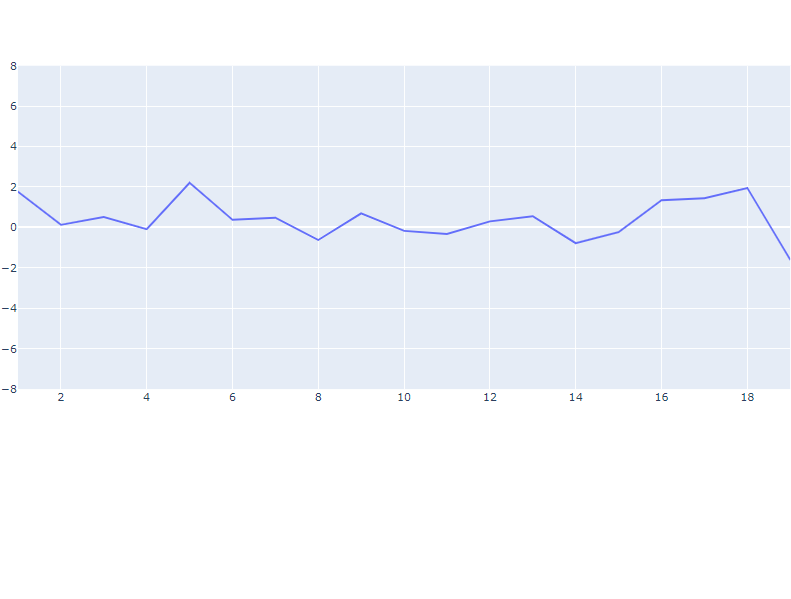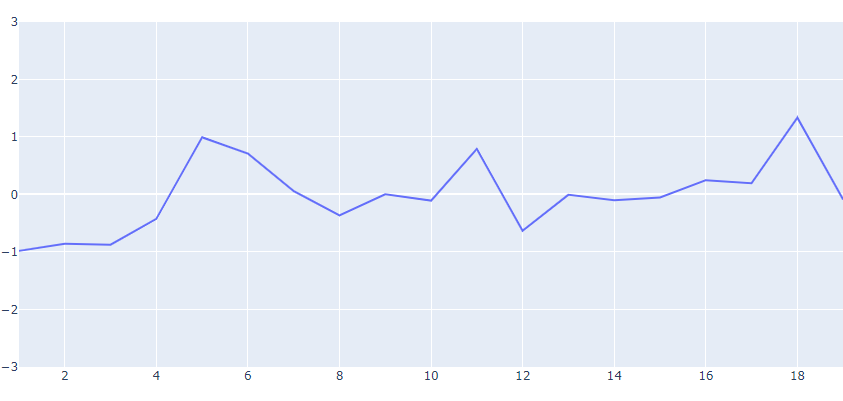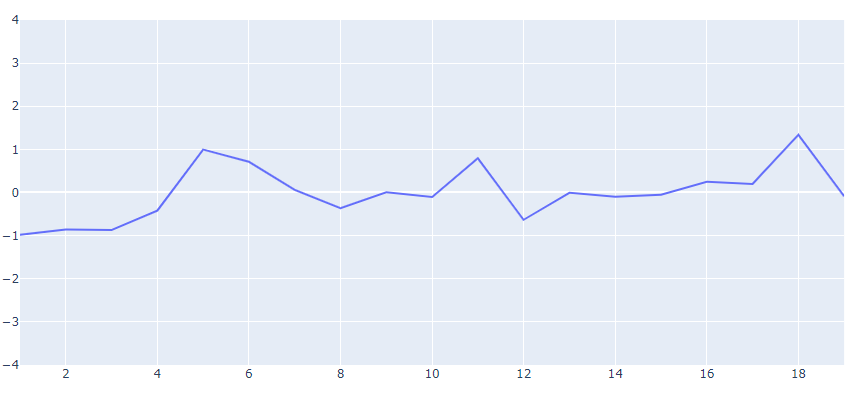Open In App

# Python Plotly: How to set the range of the y axis?

In this article, we will learn how to set the range of the y-axis of a graph using plotly in Python.

To install this module type the below command in the terminal:

`pip install plotly`

Example 1: Using layout_yaxis_range as a parameter

In this example, we have first import the required libraries i.e pandas,numpy and plotly.objs, then we generated some list of numbers of plotting on the x-axis and y-axis, Further, we have used go.Scatter() function to make a scatter plot. The go.Figure() function takes in data as input where we set the mode as ‘lines’ using mode=’lines’.We have used the magic underscore notation i.e layout_yaxis_range=[-8,8] to set the y-axis range from -8 to 8. At last we display the figure using the show() function.

## Python3

 `# Importing Libraries``import` `pandas as pd``import` `plotly.graph_objs as go``import` `numpy as np` `# generating numbers ranging from 1 to 20``# on x-axis``x ``=` `list``(``range``(``1``,``20``))` `# generating random numbers on y-axis``y ``=` `np.random.randn(``20``)` `# plotting scatter plot on x and y data with 'lines'``# as mode and setting the y-axis range from -8 to 8``fig ``=` `go.Figure(data``=``go.Scatter(x``=``x, y``=``y, mode``=``'lines'``),``                ``layout_yaxis_range``=``[``-``8``,``8``])` `# to display the figure in the output screen``fig.show()`

Output:Example 2: Using update_layout() function to set the y-axis range later

In the following example, Here we have built the plot without setting the y-axis range at first.Further, we set the y-axis range using update_layout() function i.e fig.update_layout(yaxis_range=[-3,3]) to set the range from -3 to 3.

## Python3

 `# Importing Libraries``import` `pandas as pd``import` `plotly.graph_objs as go``import` `numpy as np` `np.random.seed(``5``)` `# generating numbers ranging from 1 to 20``# on x-axis``x ``=` `list``(``range``(``1``,``20``))` `# generating random numbers on y-axis``y ``=` `np.random.randn(``20``)` `# plotting scatter plot on x and y data with``# 'lines' as mode``fig ``=` `go.Figure(data``=``go.Scatter(x``=``x, y``=``y, mode``=``'lines'``))` `# setting the y-axis range from -3 to 3``fig.update_layout(yaxis_range``=``[``-``3``,``3``])` `# to display the figure in the output screen``fig.show()`

Output:Example 3:

Similarly here we passed dict(range=[-4,4]) as a dictionary of numbers to parameter yaxis inside update_layout() function.

## Python3

 `# Importing Libraries``import` `numpy as np``import` `pandas as pd``import` `plotly.graph_objs as go` `np.random.seed(``5``)` `# generating numbers ranging from 1 to 20``# on x-axis``x ``=` `list``(``range``(``1``,``20``))` `# generating random numbers on y-axis``y ``=` `np.random.randn(``20``)` `# plotting scatter plot on x and y data with``# 'lines' as mode``fig ``=` `go.Figure(data``=``go.Scatter(x``=``x, y``=``y, mode``=``'lines'``))` `# and setting the y-axis range from -4 to 4``fig.update_layout(yaxis``=``dict``(``range``=``[``-``4``,``4``]))` `# to display the figure in the output screen``fig.show()`

Output: